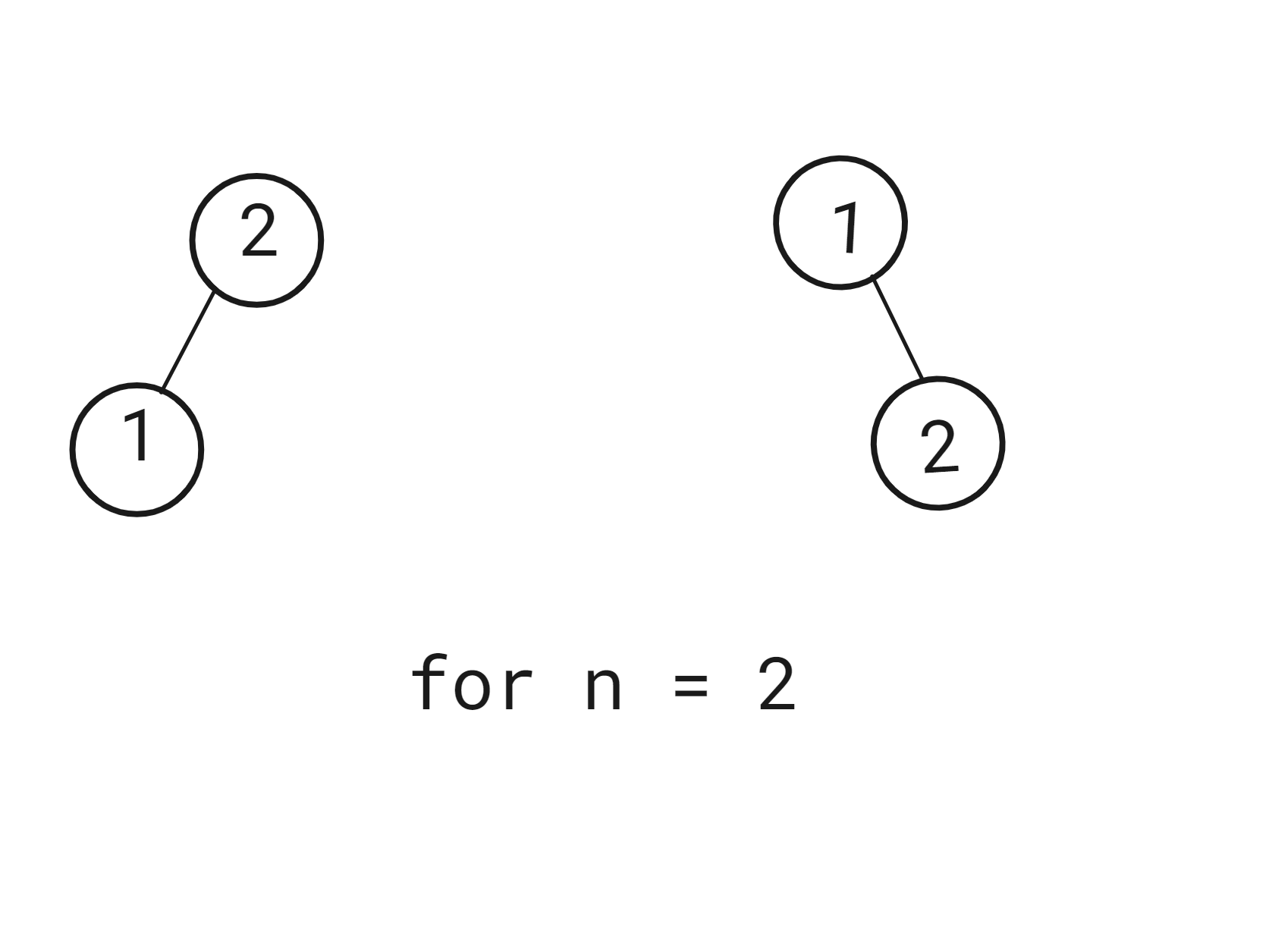New update is available. Click here to update.
Last Updated: 23 Mar, 2021
##### Unique Binary Search Trees
Moderate
Problem statement#### For example:

``````Given  ‘N’ = 2, The total number of BST’s is 2.
``````#### Note:

``````1. A binary search tree is a rooted binary tree whose internal nodes each store a key greater than all the keys in the node's left subtree and less than those in its right subtree.

2. A structurally unique binary search tree is a tree that has at least 1 node at a different position or with a different value compared to another binary search tree.
``````

#### Input format:

``````The first line of input contains an integer T denoting the number of test cases.

The first and the only line of each test case contains an integer 'N', the number of ‘nodes’.
``````

#### Output format :

``````For each test case, print a single line containing a single integer denoting the total number of BST’s that can be formed. The output of each test case will be printed in a different line.

The output of each test case will be printed in a separate line.
``````

#### Note :

``````You don't have to print anything. It's been already taken care of. Just implement the given function.
``````

#### Constraints:

``````1 <= T <= 25
1 <= N <= 30

Where ‘T’ is the total number of test cases, and N is the number of nodes.

Time limit: 1 sec.
``````Approaches

## 01ApproachThe main idea is to calculate all possible configurations using recursion.

• Let numTrees( i ) denote the number of nodes on the left side of the root.
• That implies numTrees(n - i - 1) denotes the number of nodes on the right side of the root.
• Hence the total number of BSTs possible will be : numTrees(i) * numTrees(n -  i - 1) for a given root.
• Total number of BSTs possible will be : n * numTrees(i) * numTrees(n-i-1) , where n is number of different root configurations.
• Therefore loop from 1 to n and for every ‘i’ add numTrees(i) * numTrees(n-i-1) to the answer.